### What is data visualization

Data visualization is an act of representing data or information using visual elements such as maps or graphs. The main aim is to communicate  clearly and efficiently to the audience. It is a means to aggregate a plethora of data elements at a glance and an important step in exploratory data analysis. A pictorial or graphical representation of data helps decision makers to easily see or identify new patterns or trends.

One can represent data visually using area chart ,bar chart, histogram, pie chart, line chart, box-and-whisker , cloud, bullet graph, cartogram, circle view, dot distribution map, gantt chart, heat map and more.

### Variable (data type) Classification

There are broadly two classes of data; categorical data and numerical data. Different visual elements are suitable for different data types. Here, a brief look is made into the different types of data and the visual elements used in representing them.

• Categorical data

Categorical data, as the name implies, is a collection of information that is divided into groups. That is, qualitative variables take on values in one of K different classes, or categories called the cardinality of the categorical variable. There are two types of categorical data; ordinal and nominal data. While nominal data has no order(e.g male, female), ordinal data have order (e.g very small, small, large, very large).Bar charts, pie charts and frequency tables are suitable for representing categorical data.

• Numerical data

Numerical data is a data type expressed in numbers, rather than natural language description. Sometimes called quantitative data, numerical data is always collected in number form. There are numerous tools that can be used to represent numerical data. These include; dot plots, stem and leaf graphs, histograms, box plots, ogive graphs, and scatter plots. As part of exploratory data analysis, plot of correlation among numerical data variables would help to determine the relationship among the variables.

### Basic R Plots

We can plot many basic graphs in R without the need of any extra packages. However, it may be difficult to graph certain plots. Most often, the plots are of low quality. Let’s see how it works.

• Scatterplot: The `plot()` function is used to graph scatterplot in R. The following example illustrates how to plot a scatterplot;

Code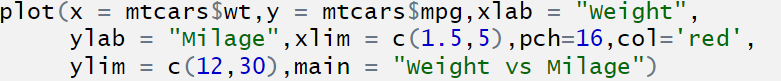Output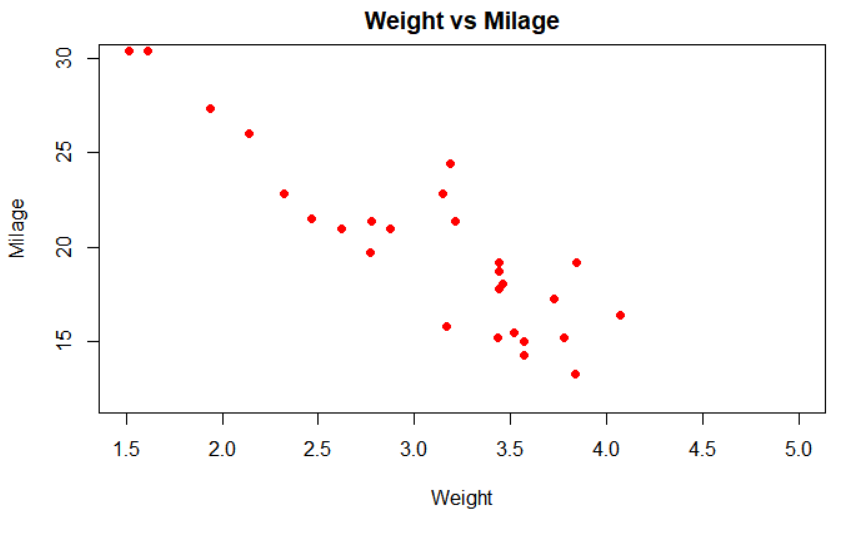• Histogram:` hist() `function is used to create histograms in R.

CodeOutput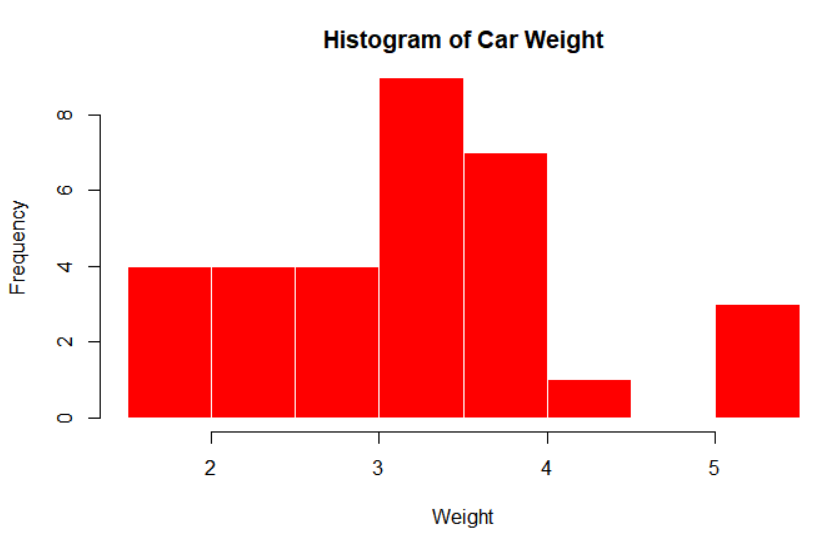• Bar chart:` barplot()` function is used to create bar charts in R.

code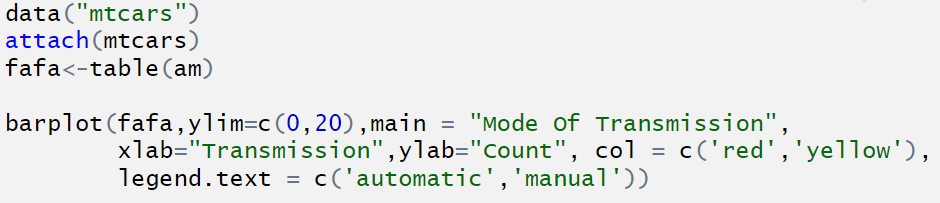Output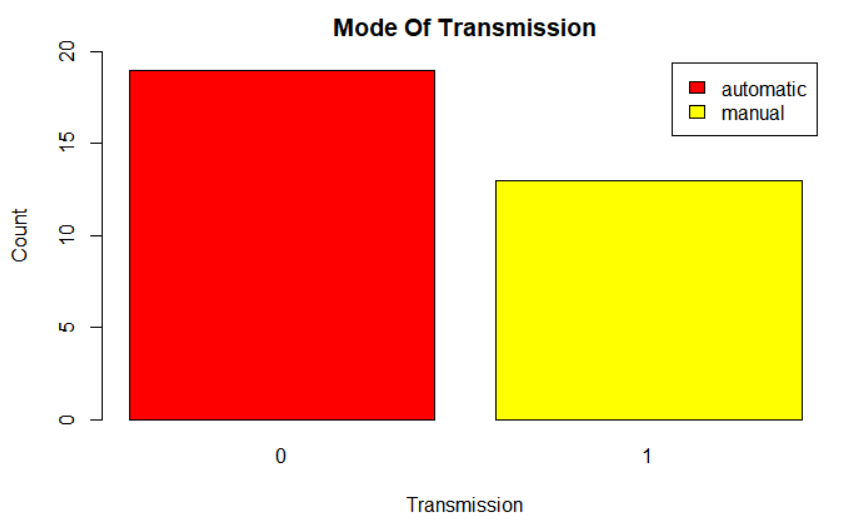• Boxplot

Boxplots are created in R by using the `boxplot()` function.

Code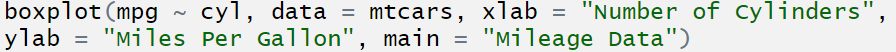Output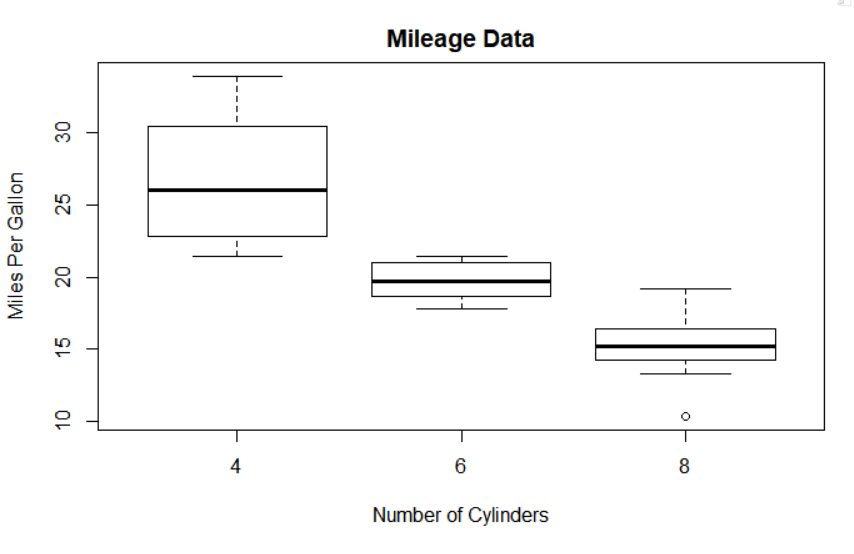The `plot() `function can also be used with the x-axis taking a categorical variable to create boxplots. `as.factor() `has been used to convert the number of cylinders (cyl) variable to categorical.

### Important data visualization packages in R

The following are some important packages used for data visualization in R; ggplot2,Lattice,highcharter,Leaflet,RColorBrewer, Plotly, sunburstR, RGL dygraphs etc.

Here, R will be used to do some basic plots and explain at a superficial level how lattice and ggplot2 work.

#### ggplot2

ggplot2 is a powerful and flexible R package, implemented by Hadley Wickham. It creates elegant data visualizations using the grammar of graphics. The “gg” in ggplot is from the grammar of graphics. It is one the top data visualization packages in R. This package is designed to work in a layered fashion, starting with a layer showing the raw data then adding layers of annotations and statistical summaries. It uses faceting to divide a plot into a matrix of panels. Each of these panels shows a different subset of the data. The basic components of a ggplot2 plot are;(1) data, (2) Aesthetics and (3) Geometry.

There are basically two functions in the ggplot2 package, namely; `qplot()` for simple plot and `ggplot()` which is more robust. Basically, one starts with one of these functions and supplies a dataset and aesthetic mapping  (with `aes()`). Then add layers (like `geom_point() `or `geom_histogram()`), scales (like `scale_colour_brewer()`), faceting specifications (like `facet_wrap()`) and coordinate systems (like `coord_flip()`).  It is worth noting that ggplot is a function while ggplot2 is a package. Let’s look at a few plots using  the ggplot2 package. Here, the qplot and ggplot functions are used to do basic data visualizations. We will be using the iris dataset for most of the plots.

Load the ggplot2 package and attach the iris dataset to expose its variables for easy use.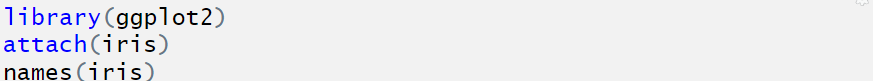• Scatterplot

Code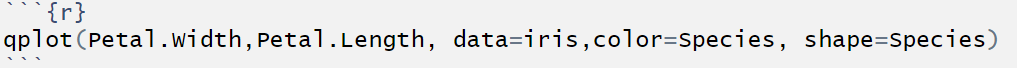Output is as follows;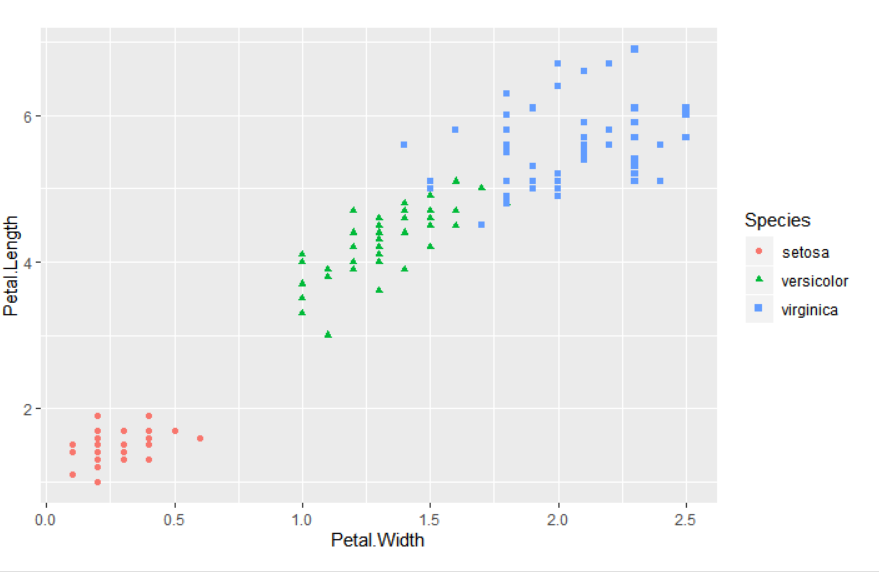• Line chart

Let’s generate data and use for the line chart.

Code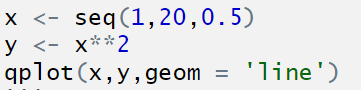Output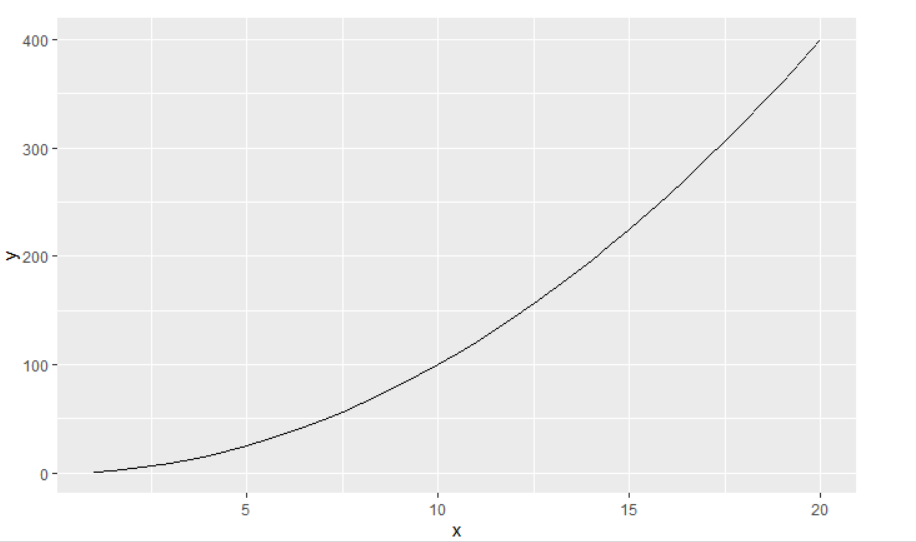Let’s use a survey data to show trend of genus over time (time series). This dataset can be found here

Code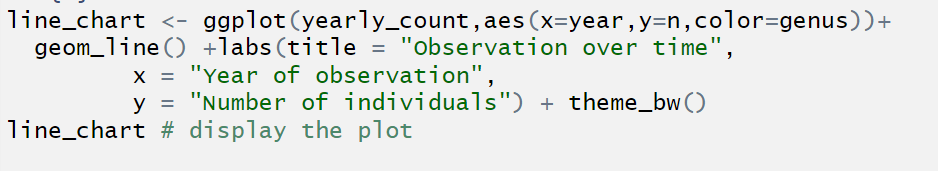Output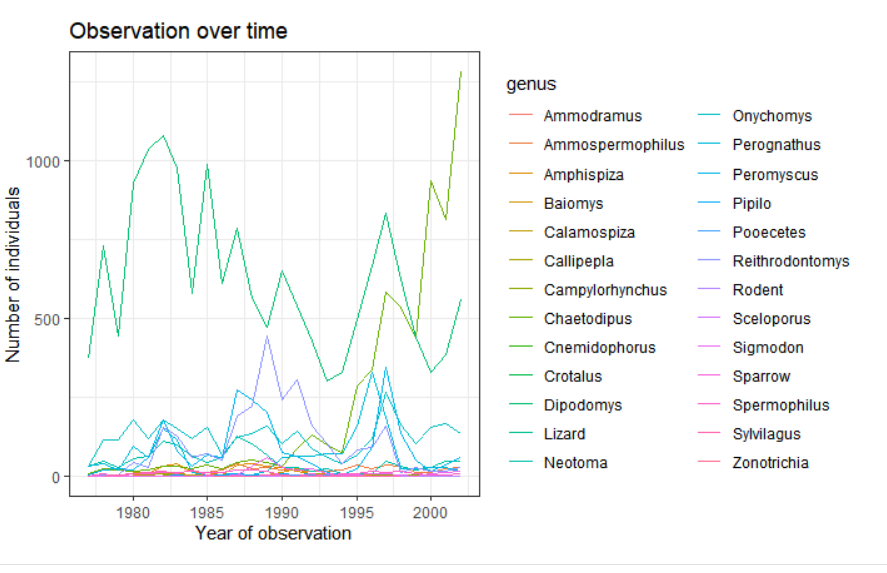• Boxplot

In the following code,` geom='boxplot'` has been used to specify that we want a boxplot in the `qplot()` function. If `ggplot()` function were used, the` geom_boxplot()` layer would have been used .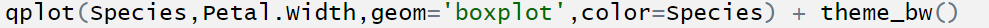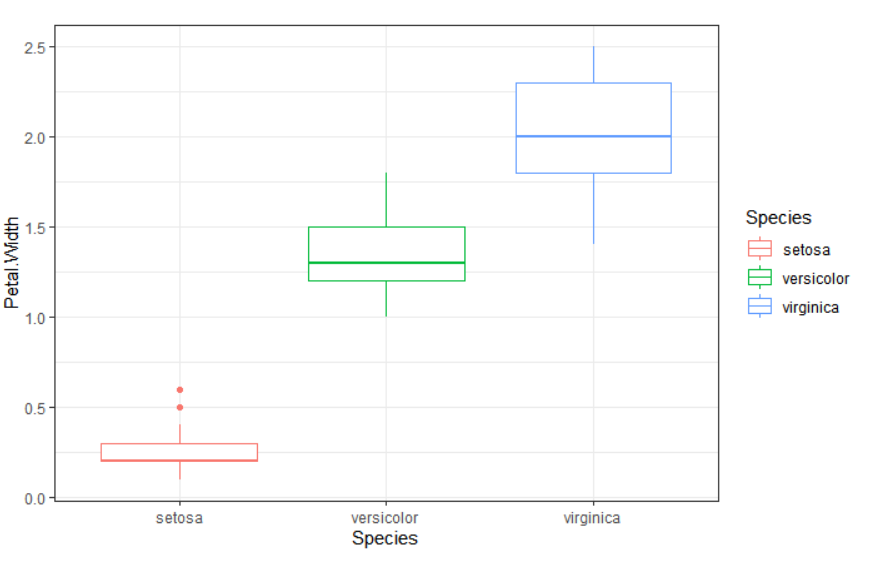• Histogram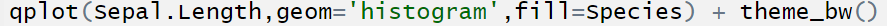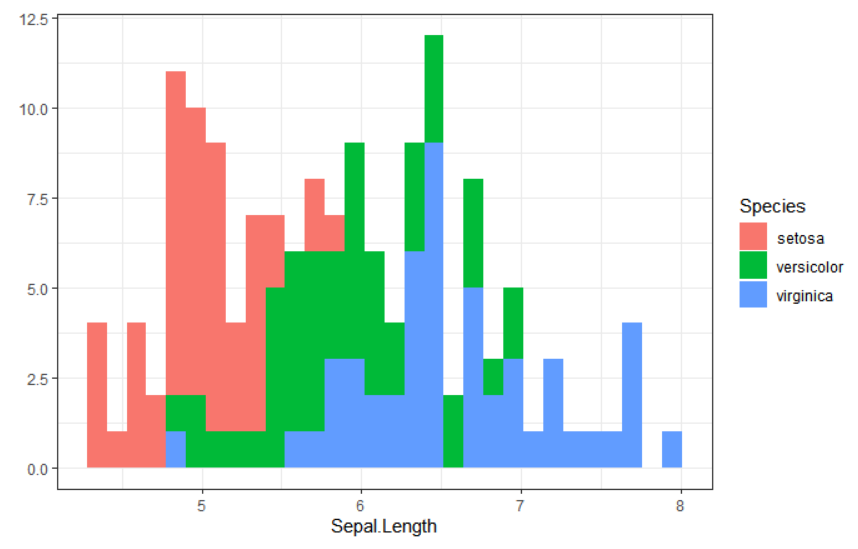Faceting using ggplot. Notice that this is done by simply adding the `facet_wrap` layer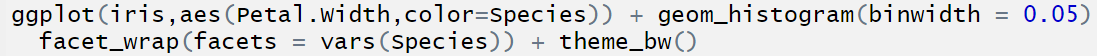Notice how this has produced multiple subplots.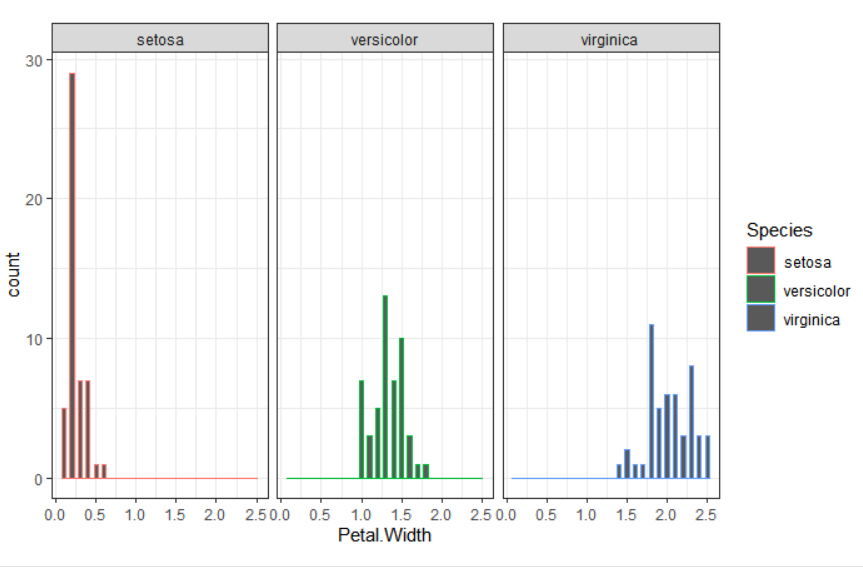• Bar chart

This done using the geom_bar() layer.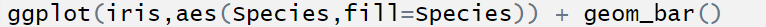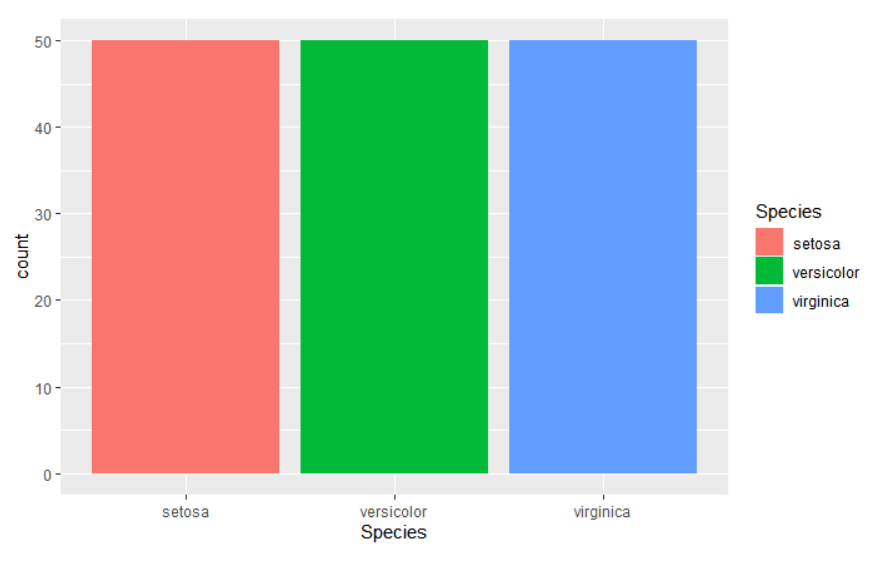#### Lattice

It is a powerful and elegant high-level data visualization system with an emphasis on multivariate data. It is designed to meet most typical graphics needs with minimal tuning, but can also be easily extended to handle most nonstandard requirements. Let’s look at some basic plots in Lattice.

This illustration is  meant to show us how the use lattice to make great plot with few lines of code

Let’s get some summary of the dataset. It has 32 data points (observations or data elements).Notice that most of the columns are numeric. However, some of these attributes are categorical.To use them efficiently, it is of utmost importance to convert them to factor so that R can interpret them as categorical variables. You can get more info about this dataset using `?mtcars`.

We will convert the number of carburetors (carb) and the transmission (am) into factor since they are categorical variables.

• Scatterplot

Output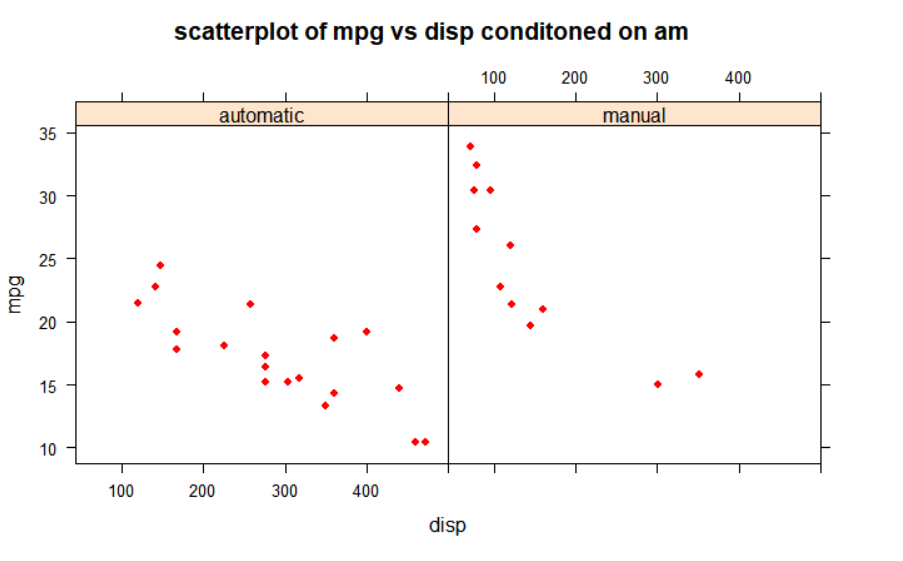It is easy plot a matrix of scatter plot using the splom() as follows;

Output

Notice that the anti diagonal divides the plots into two identical parts and contains the name of the variables. The scatter plot matrix shows how the variable is related to each other. It is an important plot to see the correlation between variables. The first plot (top-right) shows the relationship between mpg and wt, the 4th cell has the plot between draft and wt,the 16th cell holds a plot of mpg against disp and so on.

• Boxplot

Boxplot of wt grouped by the number of carburetors

output

• Histogram

Output

General note

• Histogram and box plot are suitable for visualizing a single continuous variable.
• Scatterplot is best for two continuous variables
• Boxplot can also be used for one continuous variable and one categorical variable.

Resouces